﻿ Motion Planning of Mobile RobotPublications are Open
Access in this journal
Article Versions
Export Article
• Normal Style
• MLA Style
• APA Style
• Chicago Style
Research Article
Open Access Peer-reviewed

### Motion Planning of Mobile Robot

Tomáš Lipták , Michal Kelemen, Alexander Gmiterko, Ivan Virgala, Darina Hroncová
Journal of Automation and Control. 2017, 5(2), 54-59. DOI: 10.12691/automation-5-2-5
Published online: December 11, 2017

### Abstract

The article deals with the issue of mathematical modelling of wheeled mobile robot. The introductory part of article contains theory regarding different approaches of mathematical modelling that we used. Further we applied these methods of mathematical modelling to wheeled mobile robot and we determined the mathematical model. The last part contains the motion planning in the base space and motion planning in the fiber space. These simulations of movement were realized in MATLAB.

### 1. Introduction

A fundamental robotics task is to plan collision-free motions for complex bodies from a start to a goal position among a collection of static obstacles. Although relative simple, this geometric path planning problem is provably computationally hard 1. Extensions of this formulation take into account additional constraints that are inherited from mechanical and sensor limitations of real robots such as uncertainties, feedback and differential constraints, which further complicate the development of automated planners. Modern algorithms have been fairly successful in addressing hard instances of the basic geometric problem and a lot of effort is devoted to extend their capabilities to more challenging instances. These algorithms have had widespread success in applications beyond robotics, such as computer animation, virtual prototyping, and computational biology. There are many available surveys 2, 3, 4 and books 5, 6, 7 that cover modern motion planning techniques and applications.

In this paper, we limit our motion planning analysis to a specific class of nonholonomic systems, namely Chaplygin systems. This class of systems has symmetry and is referred to as purely kinematic in 8 or principally kinematic systems in 9. Moreover, for this class of systems, the nonholonomic constraints annihilate all the generalized momentum variables. In other words, for such systems, there exists the right number of linearly independent nonholonomic constraints that completely specify the motion of the system. The body of literature relating to the mechanics of nonholonomic systems is enormous. Symmetry and reduction of such system, controllability, and time-optimal trajectories of such system is a short list of topics that pertain to nonholonomic system. Extensive overviews of nonholonomic systems can be found in 8 and 10. In this paper, we will limit ourselves to the motion planning problem of nonholonomic systems, and specifically to the principally kinematic subclass of systems.

In this paper, we propose a different approach to the motion planning problem of principally kinematic systems. For a given trajectory in the fiber space we solve analytically for the gait that locomotes the system along the desired trajectory.

### 2. The Mathematical Modelling

2.1. Lagrangian Mechanics

When we work with mechanical systems, we will assume the existence of Lagrangian function. In the absence of constraints, dynamic equations can be derived from Lagrange’s equations 11:(1)

where τi is input torque. In general apply, that some type of interaction with environment we will model as a constraint. We will devote to constraints, which are linear in velocities. Let we have k constraints, which we can write as set of k vector equations: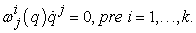(2)

This class of constraints includes most commonly investigated nonholonomic constraints. The constraints can be incorporated into the dynamics through the use of Lagrange multipliers λ. That is, equation (1) is modified by adding a force of constraint with an unknown multiplier λ: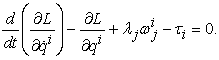(3)
2.2. Geometric Mechanics

Geometric mechanics represents the application of differential geometry to problems in classical mechanics. When studying locomotion systems, it is important to note two additional characteristics that can be used to simplify the equations of motion.

First, the configuration spaces of these systems can be decomposed into the structure 11: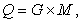(4)

where G is Lie group (often used to describe position and orientation) and M is any manifold (often called the shape space, as it is used to describe the internal shape of a robot). In many cases, the shape space is assumed to be controlled by suitable inputs. The calculation of the reduced equations is important in justifying this assumption and designing appropriate controls.

Second, Lagrangians of these systems are invariant with respect to the group action of G. That is, these systems exhibit a symmetry. In these cases, it can be shown that the equations of motion can be put in the following simplified form: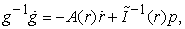(5)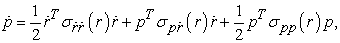(6)(7)

Equation (5) we call as reconstruction equation and express the dependence fiber velocities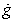on base velocitiesand momentum p. Momentum equation (6) describes evolution of momentum and reduced base dynamic equation (7) describes evolution of base variables for given generalized fiber, where M is reduced mass-inertia matrix, C contains Coriolis and centripetal force terms and N includes conservative forces and any additional external forces such as friction.

The reconstruction equation (5) and the momentum equation (6) represent set of l first order differential equations, where l is dimension of the fiber space G. The reduced base dynamic equation (7) is set of m second order differential equations, where m is dimension of the base space M. Thus, we reduce original set of n = (m + l) second order dynamic equation of motion obtained from equation (4) to set of 2l first order differential equations and m second order differential equations 11.

2.3. Comparison of Different Approaches to Mathematical Modelling

1. the mathematical model contains n second order differential equations and l first order differential equations, where n is dimension of configuration space (number of degrees of freedom) and l is number of nonholonomic constraints,

2. we do not see to the structure of differential equations from the point of view control.

1. the mathematical model contains n-l second order differential equations (reduced base dynamic equations) and l first order differential equations (reconstruction equation),

2. original differential equations were divided to two groups with following interaction – reconstruction equation does not influence to reduced base dynamic equation and is affected by this dynamic equation through torque,

3. the representation of local connection by vector fields.

2.4. Principally Kinematic Systems

Principally kinematic systems are systems that have just enough nonholonomic constraints to fully span the fiber space, that is, k = l where l is the dimension of the fiber space and k is the number of nonholonomic velocity constraints acting on the system. The reconstruction equation for principally kinematic systems has form 11: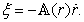(8)

### 3. Mathematical Modelling of Wheeled Mobile Robot

In following part we will show to create a mathematical model of wheeled mobile robot using Lagrangian mechanics and geometric mechanics.

• Figure 1. Wheeled mobile robot with manipulator

We will deals with the motion planning of only wheeled mobile robot. At mathematical modelling we will simplify this six wheeled mobile robot to two wheeled mobile robot because every wheel on the left rotate by the same velocityand also every wheel on the right rotate by the same velocity.

• Figure 2. The coordinates of center of mass
3.1. Lagrangian Mechanics

First, we write Lagrangian of wheeled mobile robot. We will use the form of simplified Lagrangian, where using m we refer total weight of mobile robot including the wheels, J will be moment of inertia of mobile robot around the center of mass and Jk will be moment of inertia of wheel. The simplified Lagrangian has form:(9)

Second, for wheeled mobile robot we have 3 nonholonomic constraints and we will expresses these constraints with respect to coordinate x and y: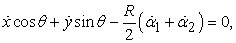(10)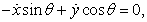(11)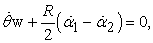(12)

where R is radius of wheel and w is half of width of mobile robot. According to equation (3) we obtain equations of motion for wheeled mobile robot:(13)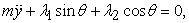(14)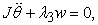(15)(16)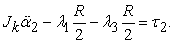(17)
3.2. Geometric Mechanics
3.2.1. Reconstruction Equation

The configuration space of mobile robot has two parts. Locally the body pose is given by (x, y, θ), where (x, y) is the location of the center of mass with respect to a global coordinate and θ is the orientation of the body with respect to the x-axis. The variables α1, α2 represent the shape, base or action variables. Thus globally the configuration space is:(18)

The mobile robot has three degrees of freedom given by variables (x, y, θ) and 2 shape (control) variables α1, α2. This means, that mobile robot belongs to under-actuated nonholonomic mechanical systems first order.

For this type of mobile robot we have 3 nonholonomic constraints, k=3 and thus we get 3 equations of motion and dimension of SE (2) group is also l=3, (l=dim{SE (2)}=3). Because of k=l=3 the mobile robot belongs to the category of principally kinematic systems, where to build the reconstruction equation fully suffice nonholonomic constraints. In this subchapter we will consider situation where the base variables represent ideal source of velocity.

At creating of the mathematical model – reconstruction equation, we will use approach where we work with nonholonomic constraints expressed in world coordinates. Nonholonomic constraints (10), (11) and (12) we will express in Pfaffian form:(19)

After multiplying of the previous equation and separating group from shape variables we obtain the reconstruction equation in the form: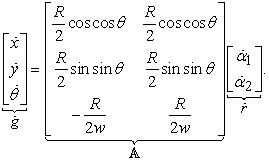(20)

Now we express reconstruction equation using local velocities:(21)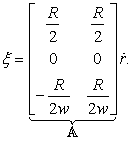(22)

3.2.2. Reduced base dynamic equation

In this subchapter we will consider situation where the base variables do not represent ideal source of velocity. The mobile robot will be controlled using torque and so we have to use also reduced base dynamic equation, whose solution are base-shape variables. Then these base variables we substitute to reconstruction equation and obtain fiber velocityor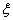.

Form of simplified Lagrangian is invariant and this special form is called as reduced Lagrangian l. This reduced Lagrangian is depend only on body velocities ξ and shape variables r,and we can express in matrix form: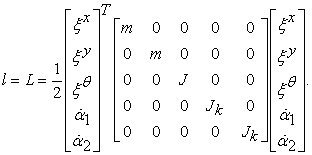(23)

Then we use relation to determine of reduced mass-inertia matrix 6: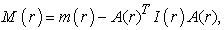(24)(25)

We will consider the terms N(r) and C(r) are equal to zero and after substituting of M(r) to equation (7) we get reduced base dynamic equations in general form:(26)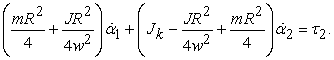(27)

When we will integrate previous equations (26), (27) and base velocities substitute to equation (22), we get desired body velocities of center of mass of mobile robot.

### 4. The Motion Planning of Wheeled Mobile Robot

At motion planning we will use only the reconstruction equation where we will consider that base variables represent ideal source of velocity, thus we will use only the reconstruction equation.

4.1. The Motion Planning in the Base Space

At this type of motion planning in the base space we design shape variables, curves, and then we analyze the shape of vector fields with shape of designed curves. We will create these curves so that we obtain maximum displacement in chosen coordinate x, y or θ.

Expressions for local connectionsare quite complicated and do not provide deep insight into the behavior of the system. However, their geometric view using visualization means provides deeper insight. One is the representation of the local connection by vector fields. Each row of the local connection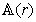can be considered as a definition of the vector field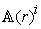on the shape (base) space whose scalar product with the shape velocity creates a corresponding component of body velocity.

The local connection of mobile robot were viewed by vector fields for R = 0.15 m and w = 0.385 m, shown in Figure 3, Figure 4 and Figure 5. Into vector fields were also at the same time generated three line segments at intervals for one second that represent the relation between the shape variables α1 and α2:

• the first segment – both wheels rotate by the same angle → α1 = α2 =,

• the second segment – both wheels rotate by the same angle with opposite orientation → α1 =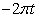, α2 =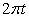→ α2 = -α1 and

• the third segment – both wheels rotate by different angle → α1 =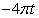, α2 =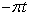→ α2 = α1/4.

• Figure 3. Vector field of local connection ξx
• Figure 4. Vector field of local connection ξy
• Figure 5. Vector field of local connection ξθ

The analysis of the shape of vector field towards the shape of generated line segments we can obtain deeper insight to the issue of the movement with nonholonomic constraints. From Figure 3, Figure 4 and Figure 5 we can deduce how mobile robot will move:

• the first line segment is parallel with the vector field of local connection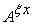and perpendicular with the vector field of local connection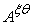, the vector field of local connectionis zero vector field → the mobile robot moves forward,

• the second line segment is perpendicular with the vector field of local connectionand parallel with the vector field of local connection, the vector field of local connectionis zero vector field → the mobile robot rotates only counterclockwise and

• the third line segment is disapprovingly oriented with the vector field of local connectionand approvingly oriented with the vector field of local connection, the vector field of local connection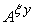is zero vector field → the mobile robot reverses and at the same time rotates counterclockwise.

The courses of individual fiber variables of mobile robot we can see in the following figure Figure 6 and in Figure 7 is shown trajectory of mobile robot in global coordinates.

• Figure 6. Courses of individual components x, y and θ
4.2. The Motion Planning in the Fiber Space

At this type of motion planning in the fiber space we need to choose curves of fiber variables and then to determine base variables. These base variables representing some curve in fiber space we substitute to the reconstruction equation. The body (local) velocities can be expressed as:(28)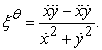(29)

When we want to follow trajectory for example in the form circle, we need to determine the terms,,,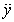:(30)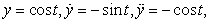(31)

then relations (28) and (29) have form:(32)(33)

Equations (32) and (33) we substitute to inverse form of reconstruction equation:(34)

and now we get relations for base variables: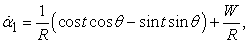(35)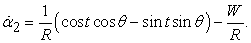(36)
• Figure 7. The trajectory of mobile robot
• Figure 8. Courses of individual components x, y and θ for desired trajectory - circle
• Figure 9. The trajectory of mobile robot

These relations of base variables (35) and (36) we substitute to the reconstruction equation and then we can simulate the movement of mobile robot. In the following figures Figure 7, Figure 8 and Figure 9. we can see block diagram, trajectory of mobile robot and courses of components x, y and θ for desired trajectory – circle.

### 5. Conclusion

The aim of paper was to introduce the use different approaches of mathematical modelling of mobile robot. At first we discussed about theory of different modelling process based on Lagrangian mechanics and geometric mechanics that we used to create mathematic model of mobile robot. In the case of Lagrangian mechanics we deduced mathematic model that consists of five second order differential equations. But in the case of geometric mechanics we obtained three first order differential equations when we consider ideal source of velocity as input. When we cannot reach ideal source of velocity we have to add two second order differential equations called as reduced base dynamic equations. The main advantage of reconstruction equation is existence of connection, where individual components of connection we can show using vector fields and then we can analyze the shape of vector fields to the shape of gait by which we obtain the ability to predict how designed system will move in the plane. The last part of article was focused on motion planning in base and fiber space. These types of motion planning was also simulated.

### Acknowledgements

The work has been accomplished under the research project No. VEGA 1/0872/16, Research of synthetic and biological inspired locomotion of mechatronic systems in rugged terrain”.

### References

  J. H. Reif. Complexity of the mover’s problem and generalizations. In Proceedings IEEE Symposium on Foundations of Computer Science, pages 421-427, 1979. In article View Article  H. H. Gonzalez-Banos, D. Hsu, and J. C. Latombe. Automous Mobile Robots: Sensing, Control, Decision-Making and Applications, chapter Motion Planning: Recent Developments. CRC Press, 2006. In article View Article  S. R. Lindemann and S. M. LaValle. Current issues in sampling-based motion planning. In P. Dario and R. Chatila, editors, Robotics Research: The Eleventh International Symposium, pages 36-54. Springer-Verlag, Berlin, 2005. In article View Article  J. T. Schwartz and M. Sharir. A survey of motion planning and related geometric algorithms. Artificial Intelligence Journal, 37: 157-169, 1988. In article View Article  H. Choset, K. M. Lynch, S. Hutchinson, G. Kantor, W. Burgard, L. E. Kavraki, and S. Thrun. Principles of Robot Motion: Theory, Algorithms, and Implementations. MIT Press, Cambridge, MA, 2005. In article View Article  J.-C. Latombe. Robot Motion Planning. Kluwer, Boston, MA, 1991. In article View Article  S. M. LaValle. Planning Algorithms. Cambridge University Press, Cambridge, U.K., 2006. Also available at http://planning.cs.uiuc.ed/. In article View Article  PubMed  J. Cort´es Monforte, Geometric, control, and numerical aspects of nonholonomic systems. Berlin; Heidelberg: Springer, 2002, vol. 1793. In article View Article  Shammas, H. Choset, and A. A. Rizzi, “Geometric motion planning analysis for two classes of underactuated mechanical systems,” The International Journal of Robotics Research, vol. 26, no. 10, pp. 1043-1073, 2007. In article View Article  A. Bloch, Nonholonomic mechanics and control. New York: Springer, c2003., vol. v. 24. In article View Article  OSTROWSKI J. P.: The Mechanics and Control of Undulatory Robotic Locomotion [thesis]. California Institute of Technology Pasadena, September 19, 1995. In article View Article

Published with license by Science and Education Publishing, Copyright © 2017 Tomáš Lipták, Michal Kelemen, Alexander Gmiterko, Ivan Virgala and Darina HroncováThis work is licensed under a Creative Commons Attribution 4.0 International License. To view a copy of this license, visit http://creativecommons.org/licenses/by/4.0/